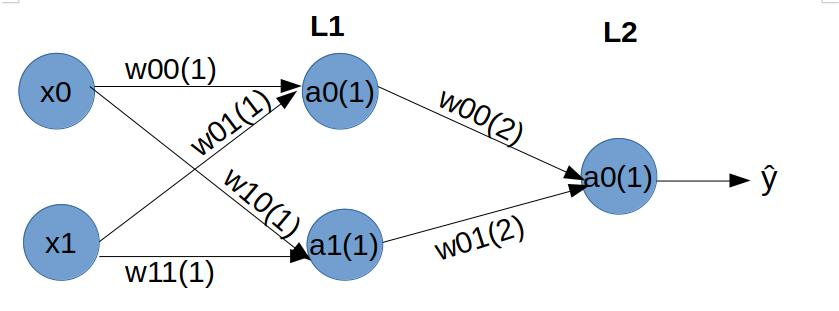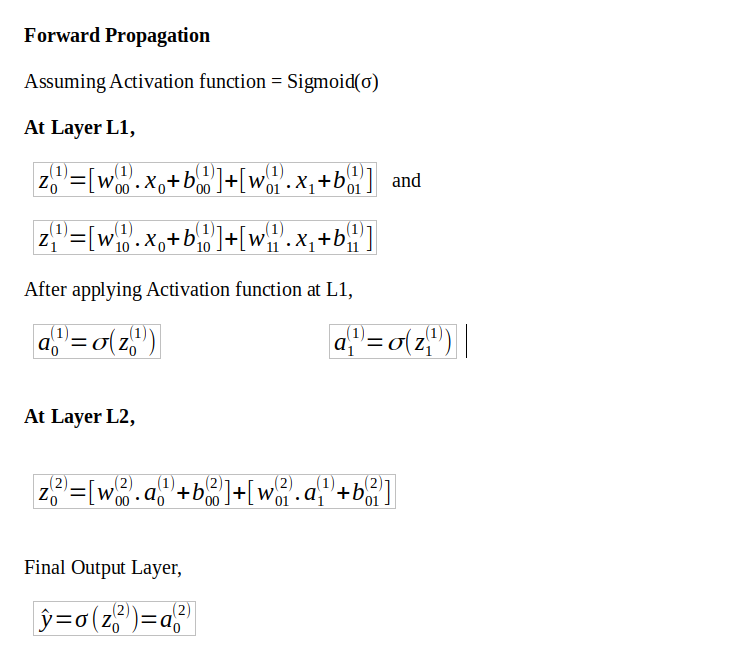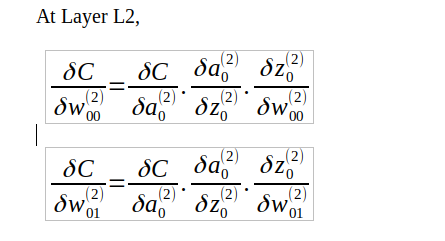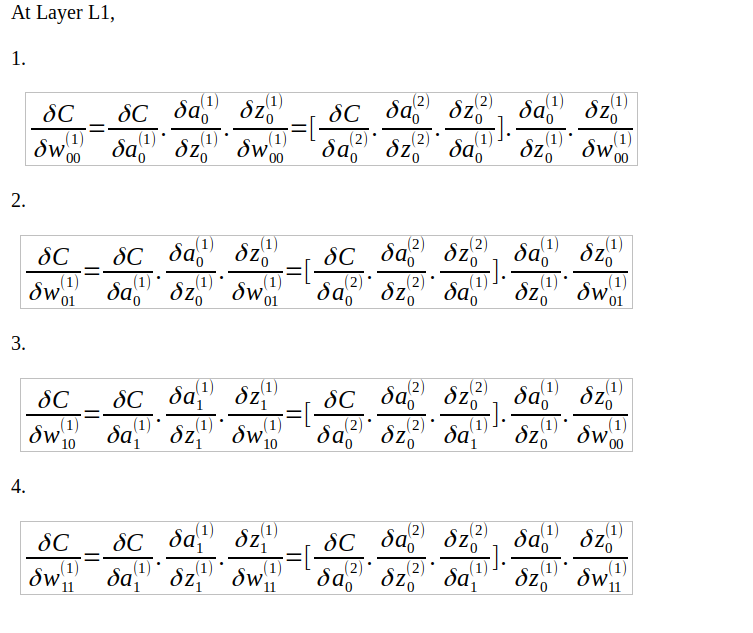# How does forward propagation and backpropagation work in deep learning?

Q:
How does forward propagation and backpropagation work in deep learning?

## ▼ Show 1 Answer

0 votes

Now, this can be answered in two ways. If you are on a phone interview, you cannot perform all the calculus in writing and show the interviewer. In such cases, it best to explain it as such:

• Forward propagation: The inputs are provided with weights to the hidden layer. At each hidden layer, we calculate the output of the activation at each node and this further propagates to the next layer till the final output layer is reached. Since we start from the inputs to the final output layer, we move forward and it is called forward propagation
• Backpropagation: We minimize the cost function by its understanding of how it changes with changing the weights and biases in a neural network. This change is obtained by calculating the gradient at each hidden layer (and using the chain rule). Since we start from the final cost function and go back each hidden layer, we move backward and thus it is called backward propagation

For an in-person interview, it is best to take up the marker, create a simple neural network with 2 inputs, a hidden layer, and an output layer, and explain it.Forward propagation:Backpropagation:

At layer L2, for all weights:At layer L1, for all weights:You need not explain with respect to the bias term as well, though you might need to expand the above equations substituting the actual derivatives.

Learn More with Madanswer

0 votes
0 votes
0 votes
0 votes
0 votes
0 votes
0 votes